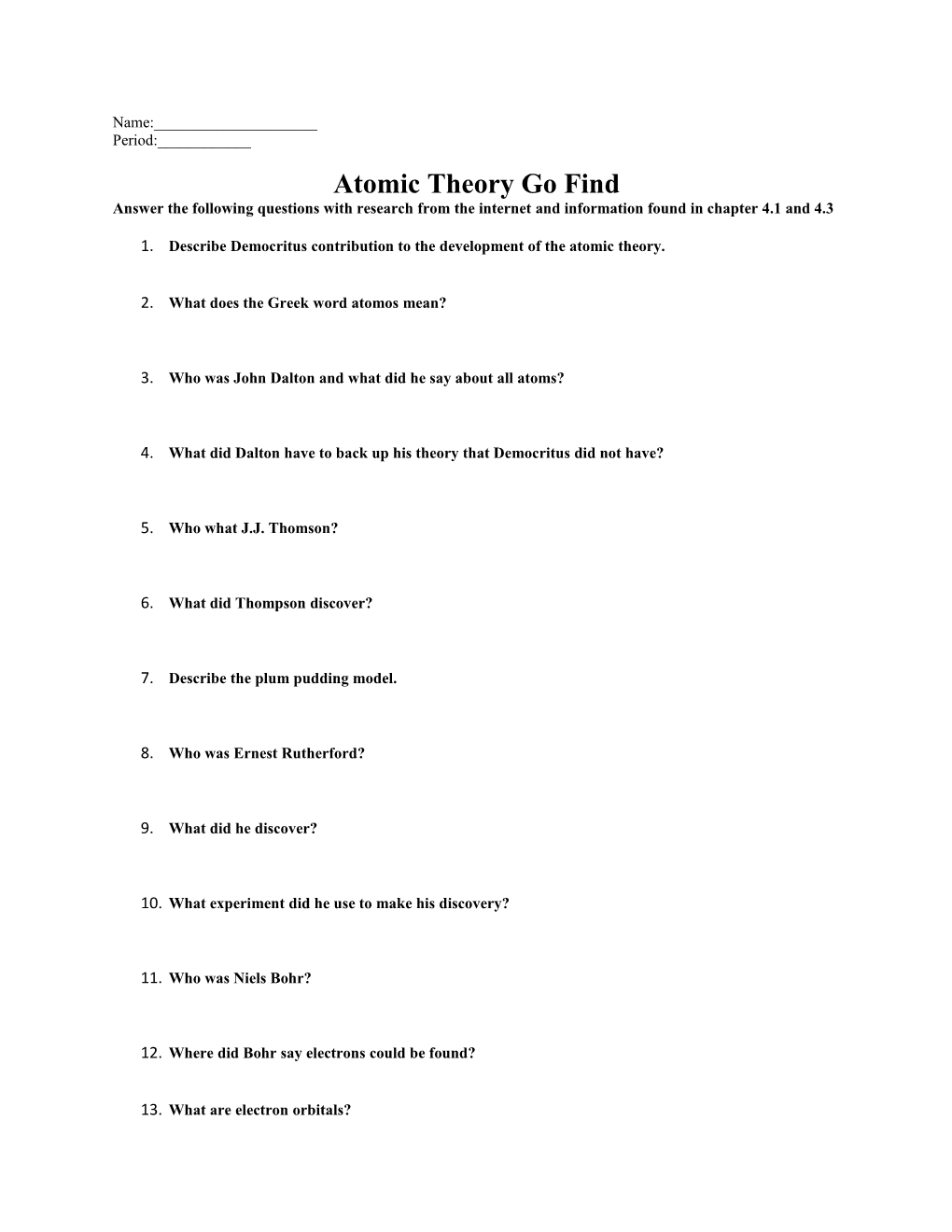# Atomic Theory Go FindName:______

Period:______

Atomic Theory Go Find

Answer the following questions with research from the internet and information found in chapter 4.1 and 4.3

1. Describe Democritus contribution to the development of the atomic theory.
1. What does the Greek word atomos mean?
1. Who was John Dalton and what did he say about all atoms?
1. What did Dalton have to back up his theory that Democritus did not have?
1. Who what J.J. Thomson?
1. What did Thompson discover?
1. Describe the plum pudding model.
1. Who was Ernest Rutherford?
1. What did he discover?
1. What experiment did he use to make his discovery?
1. Who was Niels Bohr?
1. Where did Bohr say electrons could be found?
1. What are electron orbitals?
1. What are photons and what do they have to do with electrons?

Intro to Atomic Structure Reading Guide

Answer the following questions with information found in chapter 4.2 pg 119-125.

Fill in the chart below from page 119 in your book:

Particle / Charge / Mass (kg) / Location in the atom

The 3 particles of the atom are:

a.______

b.______

c.______

Their respective charges are:

a.______

b.______

c.______

1.The atomic number tells you the number of ______in one atom of an element. It also tells you the number of ______in a neutral atom of that element.

2.The atomic number gives the “identity “of an element as well as its location on the Periodic Table. No two elements will have the ______atomic number and the atomic number of a given element never ______.

3.The ______of an element is the total number of protons and neutrons in the______of the atom.

4.The mass number is used to calculate the number of ______in one atom of an element. In order to calculate the number of neutrons you must subtract the ______from the ______.

5.An ______is an atom that has the same number of protons but a different number of neutrons relative to other atoms of the same element.

6.The ______of an element is the average mass of an element’s naturally occurring atom, or isotopes, taking into account the ______of each isotope.

7 .Give the symbol and number of protons in one atom of:

Lithium ______Bromine ______

Iron ______Copper ______

Oxygen ______Mercury ______

Krypton ______Helium ______

8.Give the symbol and number of electrons in a neutral atom of:

Uranium ______Chlorine ______

Boron ______Iodine ______

Antimony ______Xenon ______

9.Give the symbol and number of neutrons in one atom of:

(To get the “mass number”,you must round the “atomic mass ” to the nearest whole number)) Show your calculations.

Barium ______Bismuth ______

Carbon ______Hydrogen ______

Fluorine ______Magnesium ______

Europium ______Mercury ______

10.Name the element which has the following numbers of particles:

a.26 electrons,29 neutrons,26 protons ______

b.53 protons,74 neutrons ______

c.2 electrons (neutral atoms)______

d.20 protons ______

e.86 electrons,125 neutrons,82 protons (charged atom

______

f.0 neutrons ______

11.If you know only the following information can you always determine what the element is? (Yes/No).

a. number of protons ______

b.number of neutrons______

c.number of electrons in a neutral atom______

d.number of electrons______